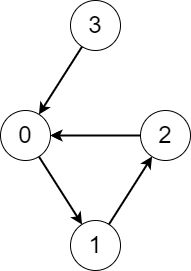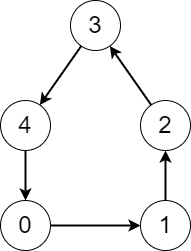# 战局详情

271 / 2909Juruoer181:21:220:04:070:14:14 10:33:48 11:11:22

# 题目及解答

100088. 有序三元组中的最大值 I

• 3 <= nums.length <= 100
• 1 <= nums[i] <= 1e6

100086. 有序三元组中的最大值 II

• 3 <= nums.length <= 1e5
• 1 <= nums[i] <= 1e6

100076. 无限数组的最短子数组

• 1 <= nums.length <= 1e5
• 1 <= nums[i] <= 1e5
• 1 <= target <= 1e9

nums 的元素和为 sum

100075. 有向图访问计数

• 你从节点 x 开始，通过边访问其他节点，直到你在 此过程 中再次访问到之前已经访问过的节点。

• n == edges.length
• 2 <= n <= 1e5
• 0 <= edges[i] <= n - 1
• edges[i] != i